# How to construct a Binary Tree using different traversals?

Problem Statement: Requirements are needed to construct a unique binary tree. In this article, we will understand the traversals required to construct a unique binary tree.

# Constructing A Unique Binary tree using a single traversal.

If we are given any traversal, be it postorder, preorder, or inorder; it is not possible to construct a unique binary tree.

For example:

For a preorder traversal: [ 1 2 3 4 5 ], all the following trees can be constructed.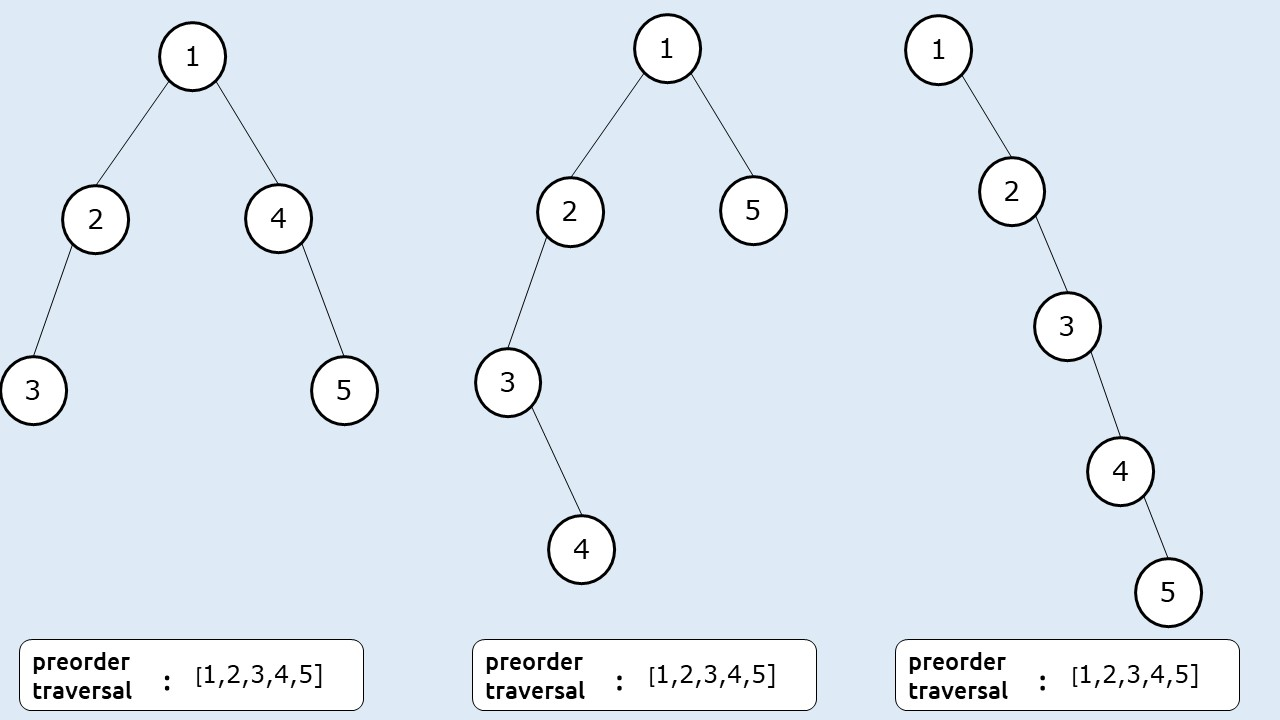For an inorder traversal : [ 1 2 3 4 5 ], all the following trees can be constructed.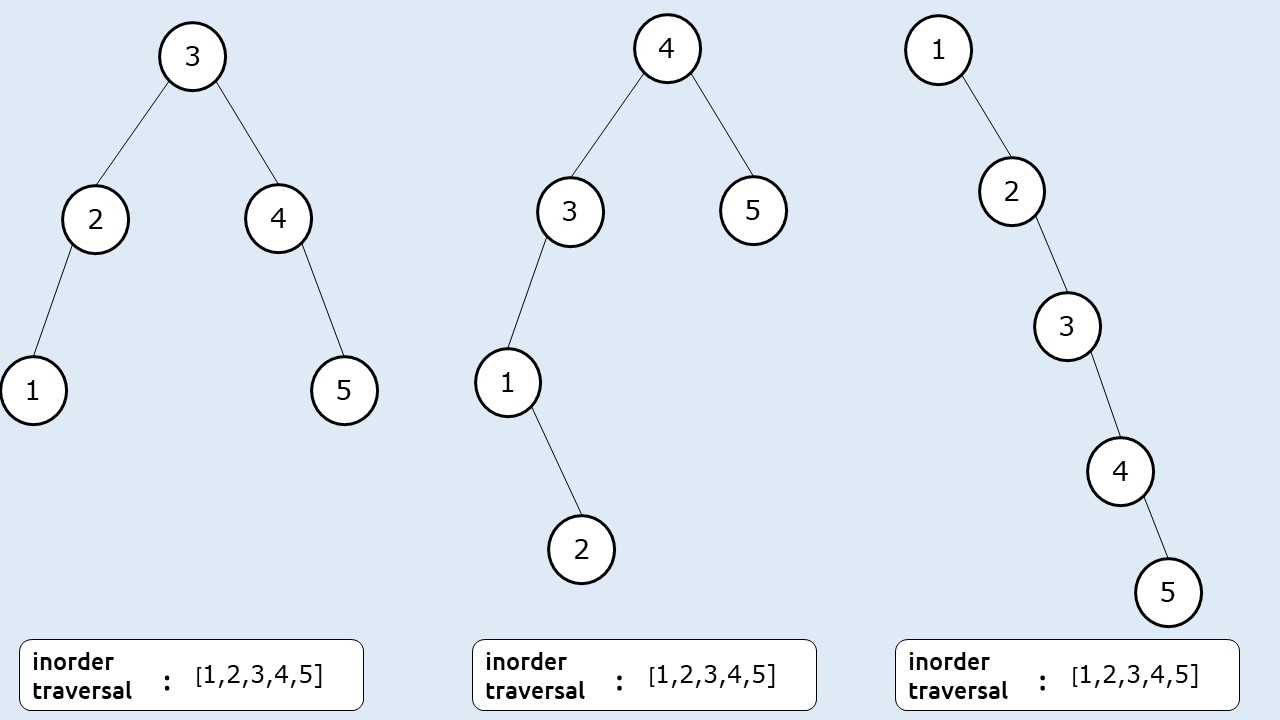Conclusion: We cannot construct a unique binary tree with just one traversal.

# Constructing A Unique Binary tree using two traversals.

Case 1: Using Preorder and Postorder Traversal

Given: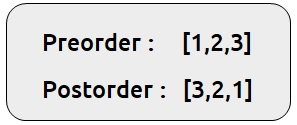We see that the following different trees can be constructed using the above two traversals: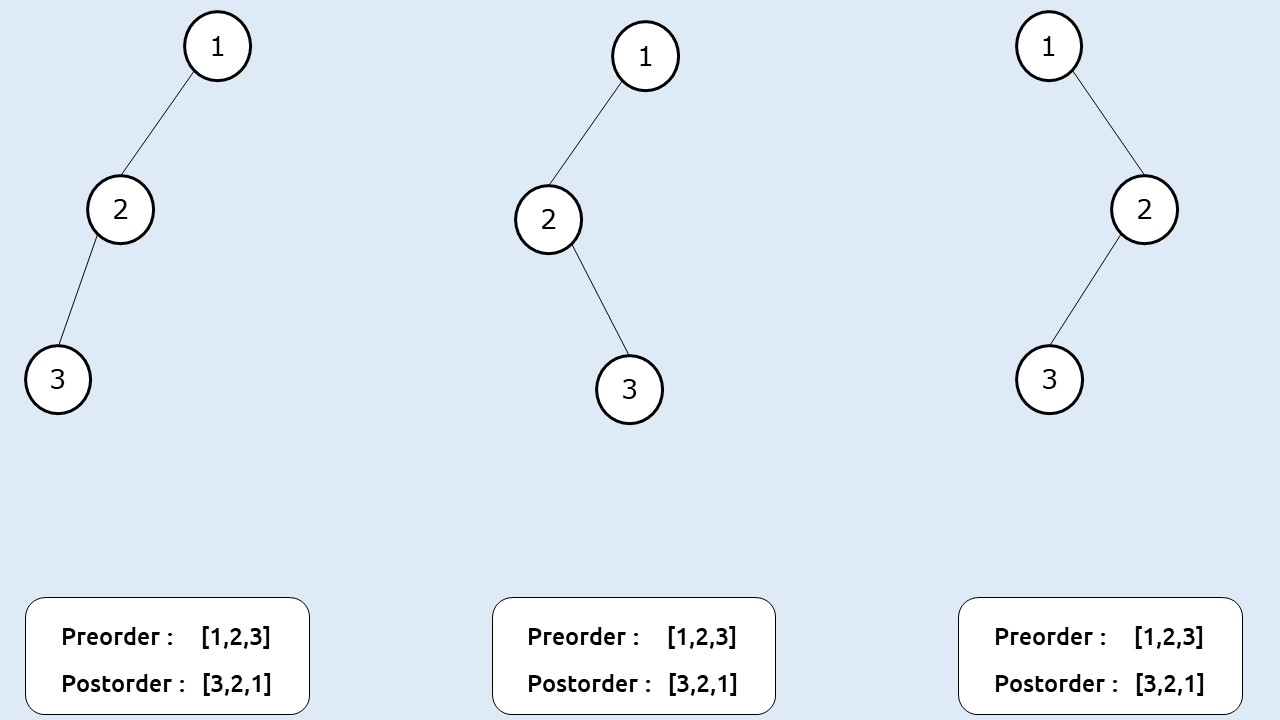Conclusion: We cannot construct a unique binary tree using preorder and postorder traversal.

Case 2: Using preorder and inorder traversal

Given: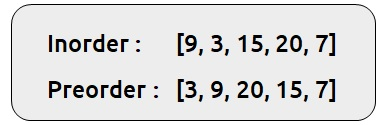We will try to construct a binary tree using the above two traversals.

Step 1:

We know that the first element of a preorder traversal (root, left, right) is the root of the tree therefore, we can say that 3 is the root of the tree. We can then find 3 in the inorder traversal and find the left subtree and right subtree of node 3 from it as inorder traversal is (left, root, right).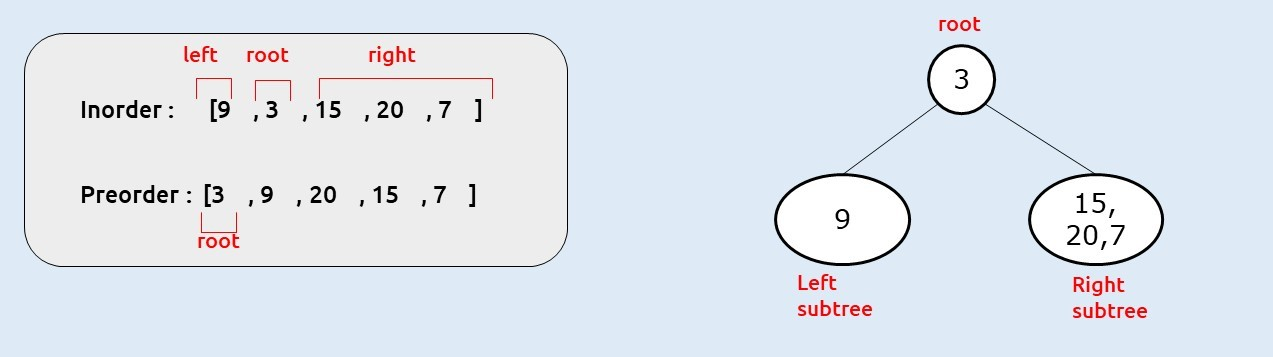Step 2:

As 9 is the only element to the left of 3 in inorder traversal, we can conclude that the left subtree of node 3 contains a single node 9. Preorder traversal also contains node 9 as the second element. Therefore the remaining nodes in the preorder traversal will be the right subtree of node 3.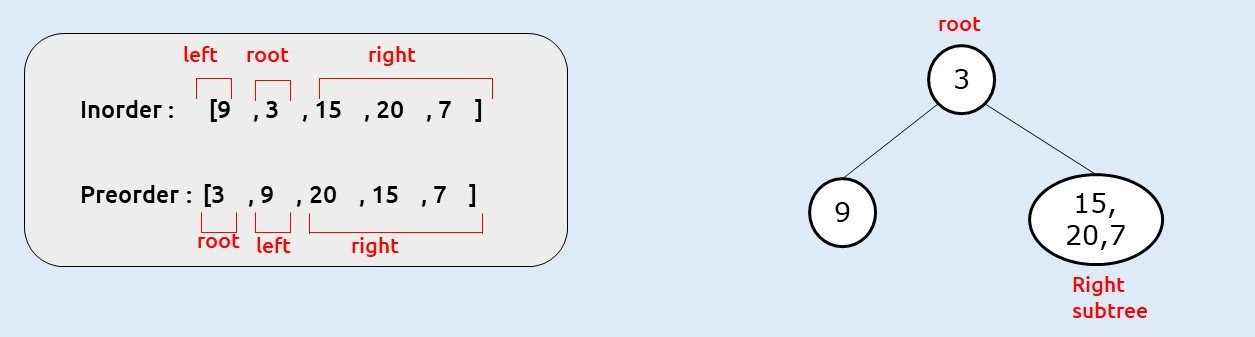Step 3:

Now, we will work on the right subtree of node 3. We know that is given by the last three elements of preorder traversal. As discussed in step 1, the first node will give us the root of the right subtree, then we can find that element (20) in the inorder traversal. Elements left to element 20 will give us the left subtree and elements right to it will give the right subtree of node 20.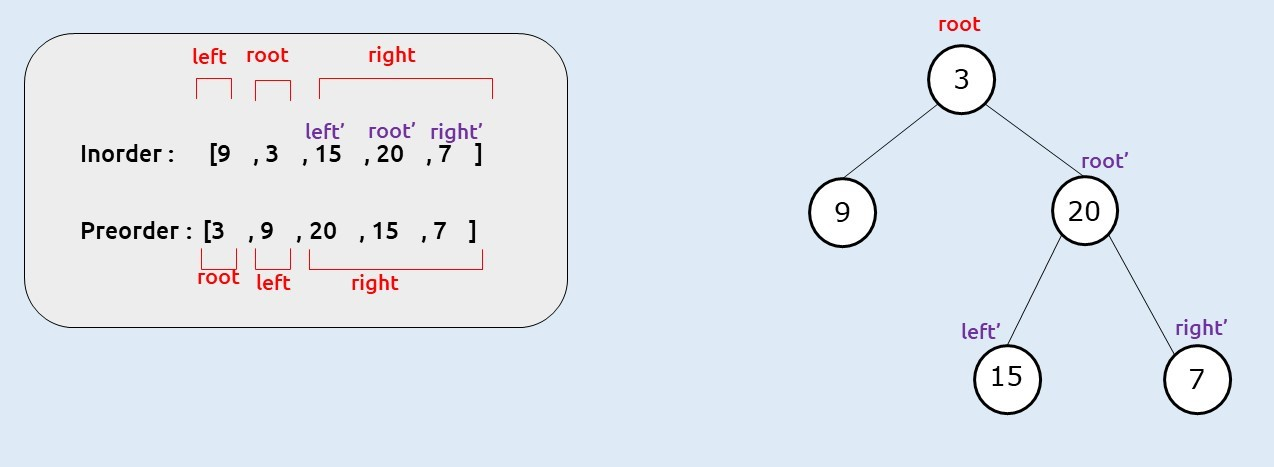Conclusion: We can construct a unique binary tree using inorder and preorder traversal.

Similarly, it can be shown that a unique binary tree can be constructed using inorder and postorder traversal as in postorder traversal the root node is given as the last element.

The special feature of the inorder traversal is that it helps us to identify a node and its left and right subtree. This is not provided by any other traversal therefore whenever we are provided in order traversal with one other traversal, we can easily construct a unique binary tree.

Key takeaways

• We cannot construct a unique binary tree using only one traversal
• We cannot construct a unique binary tree using preorder and postorder traversal.
• We can construct a unique binary tree using inorder traversal and preorder traversal or using inorder and postorder traversal.

Special thanks to Anshuman Sharma for contributing to this article on takeUforward. If you also wish to share your knowledge with the takeUforward fam, please check out this article